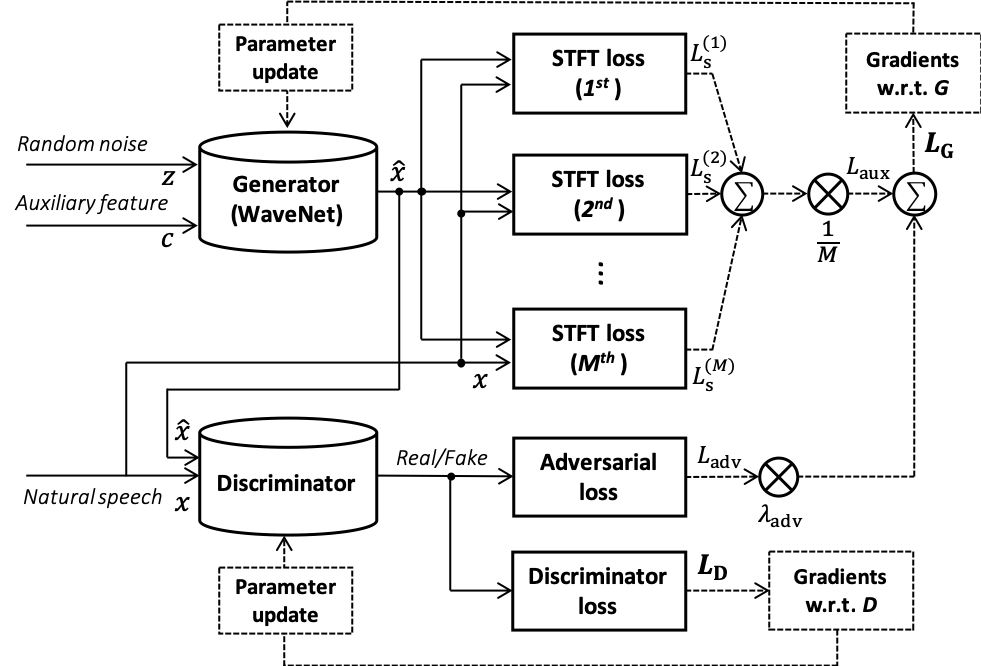# Parallel WaveGAN: A fast waveform generation model based on generative adversarial networks with multi-resolution spectrogram

Preprint: arXiv:1910.11480 (accepted to ICASSP 2020)

Japanese samples were used in the subjective evaluations reported in our paper.

## Authors

• Ryuichi Yamamoto (LINE Corp.)
• Eunwoo Song (NAVER Corp.)
• Jae-Min Kim (NAVER Corp.)

## Abstract

We propose Parallel WaveGAN1, a distillation-free, fast, and small-footprint waveform generation method using a generative adversarial network. In the proposed method, a non-autoregressive WaveNet is trained by jointly optimizing multi-resolution spectrogram and adversarial loss functions, which can effectively capture the time-frequency distribution of the realistic speech waveform. As our method does not require density distillation used in the conventional teacher-student framework, the entire model can be easily trained even with a small number of parameters. In particular, the proposed Parallel WaveGAN has only 1.44 M parameters and can generate 24 kHz speech waveform 28.68 times faster than real-time on a single GPU environment. Perceptual listening test results verify that our proposed method achieves 4.16 mean opinion score within a Transformer-based text-to-speech framework, which is comparative to the best distillation-based Parallel WaveNet system.### Systems used for comparision

• Ground truth: Recorded speech.
• WaveNet: Gaussian WaveNet 
• ClariNet-$L^{(1)}$: ClariNet  with the single STFT auxiliary loss
• ClariNet-$L^{(1,2,3)}$: ClariNet with the multi-resolution STFT loss
• ClariNet-GAN-$L^{(1,2,3)}$: ClariNet with the multi-resolution STFT and adversarial losses 
• Parallel WaveGAN-$L^{(1)}$: Parallel WaveGAN with th single STFT loss
• Parallel WaveGAN-$L^{(1,2,3)}$: Parallel WaveGAN with the multi-resolution STFT loss

## Audio samples (Japanese)

1. Analysis/synthesis
2. Text-to-speech (Transformer TTS + vocoder models)

### Analysis/synthesis

Sample 1

Ground truthWaveNetClariNet-$L^{(1)}$
ClariNet-$L^{(1,2,3)}$ClariNet-GAN-$L^{(1,2,3)}$Parallel WaveGAN-$L^{(1)}$
Parallel WaveGAN-$L^{(1,2,3)}$ (ours)

Sample 2

Ground truthWaveNetClariNet-$L^{(1)}$
ClariNet-$L^{(1,2,3)}$ClariNet-GAN-$L^{(1,2,3)}$Parallel WaveGAN-$L^{(1)}$
Parallel WaveGAN-$L^{(1,2,3)}$ (ours)

Sample 3

Ground truthWaveNetClariNet-$L^{(1)}$
ClariNet-$L^{(1,2,3)}$ClariNet-GAN-$L^{(1,2,3)}$Parallel WaveGAN-$L^{(1)}$
Parallel WaveGAN-$L^{(1,2,3)}$ (ours)

Sample 4

Ground truthWaveNetClariNet-$L^{(1)}$
ClariNet-$L^{(1,2,3)}$ClariNet-GAN-$L^{(1,2,3)}$Parallel WaveGAN-$L^{(1)}$
Parallel WaveGAN-$L^{(1,2,3)}$ (ours)

Sample 5

Ground truthWaveNetClariNet-$L^{(1)}$
ClariNet-$L^{(1,2,3)}$ClariNet-GAN-$L^{(1,2,3)}$Parallel WaveGAN-$L^{(1)}$
Parallel WaveGAN-$L^{(1,2,3)}$ (ours)

### Text-to-speech

Sample 1

Ground truthTransformer + WaveNetTransformer + ClariNet-$L^{(1,2,3)}$
Transformer + ClariNet-GAN-$L^{(1,2,3)}$Transformer + Parallel WaveGAN--$L^{(1,2,3)}$ (ours)

Sample 2

Ground truthTransformer + WaveNetTransformer + ClariNet-$L^{(1,2,3)}$
Transformer + ClariNet-GAN-$L^{(1,2,3)}$Transformer + Parallel WaveGAN--$L^{(1,2,3)}$ (ours)

Sample 3

Ground truthTransformer + WaveNetTransformer + ClariNet-$L^{(1,2,3)}$
Transformer + ClariNet-GAN-$L^{(1,2,3)}$Transformer + Parallel WaveGAN--$L^{(1,2,3)}$ (ours)

Sample 4

Ground truthTransformer + WaveNetTransformer + ClariNet-$L^{(1,2,3)}$
Transformer + ClariNet-GAN-$L^{(1,2,3)}$Transformer + Parallel WaveGAN--$L^{(1,2,3)}$ (ours)

Sample 5

Ground truthTransformer + WaveNetTransformer + ClariNet-$L^{(1,2,3)}$
Transformer + ClariNet-GAN-$L^{(1,2,3)}$Transformer + Parallel WaveGAN--$L^{(1,2,3)}$ (ours)

## Audio samples (English)

1. Analysis/synthesis
2. Text-to-speech (ESPnet-TTS + Our Parallel WaveGAN)

LJSpeech dataset is used for the test. Mel-spectrograms (with the range of 70 - 7600 Hz2) were used for local conditioning.

Please note that the English samples were not used in the subjective evaluations reported in our paper.

### Analysis/synthesis

That is reflected in definite and comprehensive operating procedures.

Ground truthWaveNetClariNet-$L^{(1)}$
ClariNet-$L^{(1,2,3)}$ClariNet-GAN-$L^{(1,2,3)}$Parallel WaveGAN-$L^{(1)}$
Parallel WaveGAN-$L^{(1,2,3)}$ (ours)

The commission also recommends.

Ground truthWaveNetClariNet-$L^{(1)}$
ClariNet-$L^{(1,2,3)}$ClariNet-GAN-$L^{(1,2,3)}$Parallel WaveGAN-$L^{(1)}$
Parallel WaveGAN-$L^{(1,2,3)}$ (ours)

That the secret service consciously set about the task of inculcating and maintaining the highest standard of excellence and esprit, for all of its personnel.

Ground truthWaveNetClariNet-$L^{(1)}$
ClariNet-$L^{(1,2,3)}$ClariNet-GAN-$L^{(1,2,3)}$Parallel WaveGAN-$L^{(1)}$
Parallel WaveGAN-$L^{(1,2,3)}$ (ours)

This involves tight and unswerving discipline as well as the promotion of an outstanding degree of dedication and loyalty to duty.

Ground truthWaveNetClariNet-$L^{(1)}$
ClariNet-$L^{(1,2,3)}$ClariNet-GAN-$L^{(1,2,3)}$Parallel WaveGAN-$L^{(1)}$
Parallel WaveGAN-$L^{(1,2,3)}$ (ours)

The commission emphasizes that it finds no causal connection between the assassination.

Ground truthWaveNetClariNet-$L^{(1)}$
ClariNet-$L^{(1,2,3)}$ClariNet-GAN-$L^{(1,2,3)}$Parallel WaveGAN-$L^{(1)}$
Parallel WaveGAN-$L^{(1,2,3)}$ (ours)

### Text-to-speech

We combined our Parallel WaveGAN with ESPnet-TTS. The systems used here are as follows:

• Transformer.v3: Transformer.v3 presented in ESPnet-TTS.
• MoL WaveNet: WaveNet with mixture of logistics output distribution (shipped with ESPnet). Pre-emphasis/de-emphasis were applied to reduce perceptual noise (similar to LPCNet).
• Parallel WaveGAN: Our Parallel WaveGAN with multi-resolution spectrogram loss.

Note that Transformer.v3 + MoL WaveNet is the same as used in https://espnet.github.io/icassp2020-tts/. Mel-spectrograms (with the range of 70 - 11025 Hz) were used for local conditioning.

That is reflected in definite and comprehensive operating procedures.

Ground truthTransformer.v3 + MoL WaveNetTransformer.v3 + Parallel WaveGAN

The commission also recommends.

Ground truthTransformer.v3 + MoL WaveNetTransformer.v3 + Parallel WaveGAN

That the secret service consciously set about the task of inculcating and maintaining the highest standard of excellence and esprit, for all of its personnel.

Ground truthTransformer.v3 + MoL WaveNetTransformer.v3 + Parallel WaveGAN

This involves tight and unswerving discipline as well as the promotion of an outstanding degree of dedication and loyalty to duty.

Ground truthTransformer.v3 + MoL WaveNetTransformer.v3 + Parallel WaveGAN

The commission emphasizes that it finds no causal connection between the assassination.

Ground truthTransformer.v3 + MoL WaveNetTransformer.v3 + Parallel WaveGAN
• W. Ping, K. Peng, and J. Chen, “ClariNet: Parallel wave generation in end-to-end text-to-speech,” in Proc. ICLR, 2019 (arXiv).
• R. Yamamoto, E. Song, and J.-M. Kim, “Probability density distillation with generative adversarial networks for high-quality parallel waveform generation,” in Proc. INTERSPEECH, 2019, pp. 699–703. (ISCA archive)

## Acknowledgements

Work performed with nVoice, Clova Voice, Naver Corp.

## Citation

@inproceedings{yamamoto2020parallel,
title={Parallel {WaveGAN}: {A} fast waveform generation model based on generative adversarial networks with multi-resolution spectrogram},
author={Yamamoto, Ryuichi and Song, Eunwoo and Kim, Jae-Min},
booktitle	= "Proc. of ICASSP",
pages={6199--6203},
year={2020}
}


1. Note that our work is not closely related to an unsupervised waveform synthesis model, WaveGAN↩︎

2. Audio quaility can be improved by using the full-band frequency range, but it may suffer from the over-smoothing problem in TTS. ↩︎